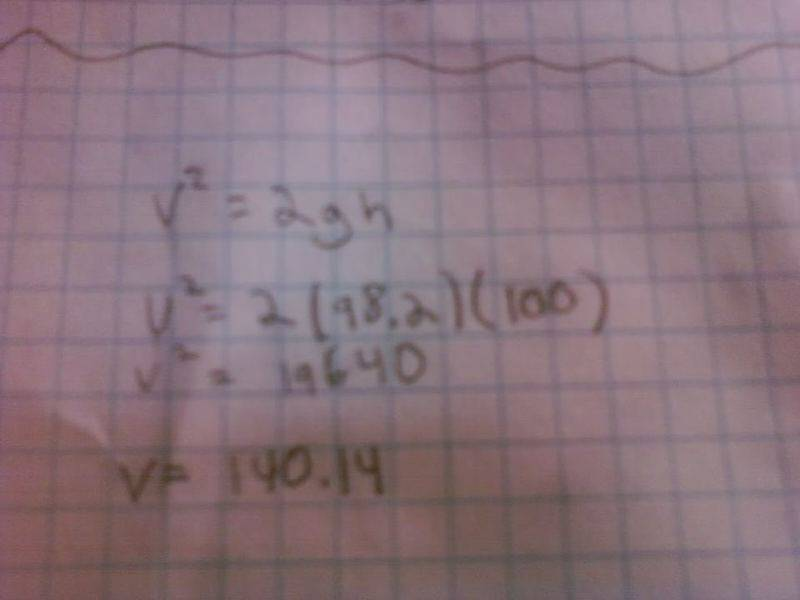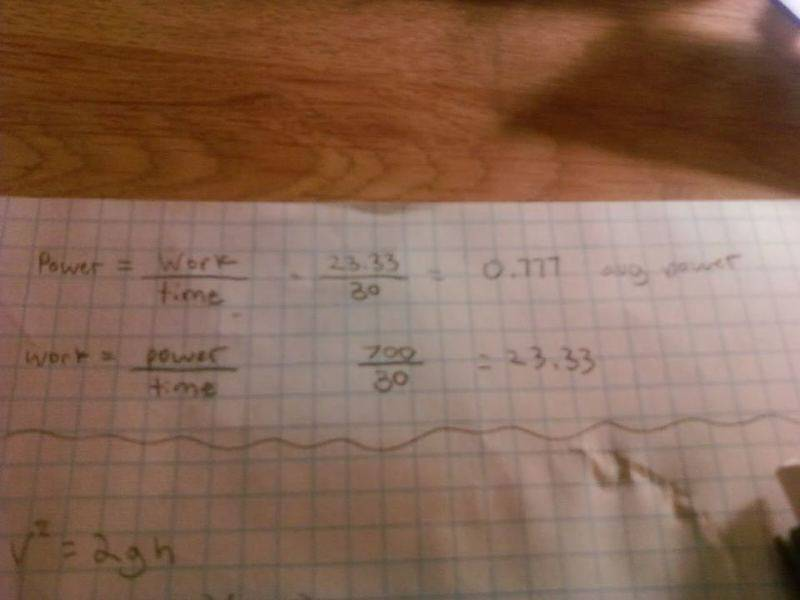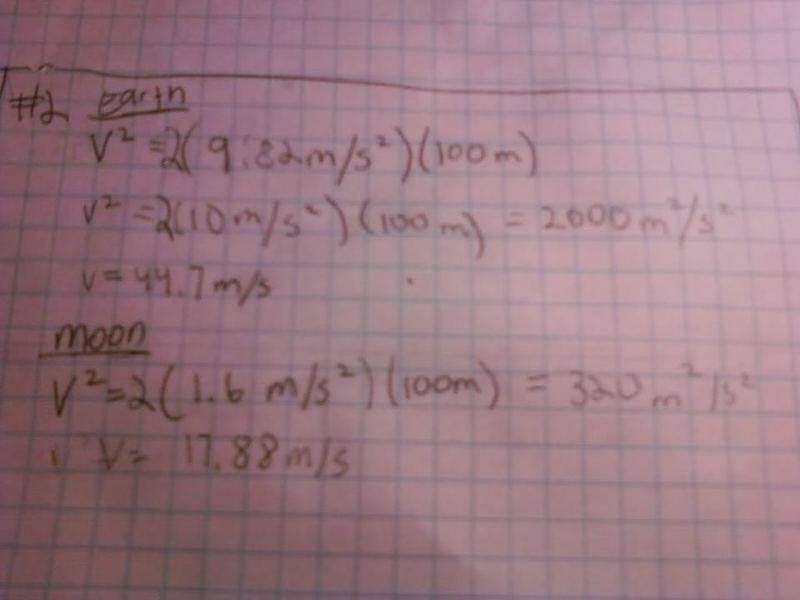# Basic Physics help ( energy )

## Homework Statement

I really need help on these two problems, I am not sure where to start and for that reason I do not have an attempt at the problem. I am not asking for anyone to do them for me , but could somone give me the equations or the steps needed to solve?

1. I expend 700 Calories (food or kcal) in riding my bicycle for 30 minutes. What was my average power during my ride?

2. NASA builds a 100 meter high roller coaster on the Moon. The gravitational force on the Moon is 1/6 that of earth. Will the roller coaster car being traveling faster at the bottom of the track on the Earth or the Moon. How much faster or slower will it be going?

## The Attempt at a Solution

Hi aforce20,
Do you remember how Power defined? It's the amount of energy used per unit time. Perhaps that will help you with the first problem.

For the second problem, are you familiar with the concept of conservation of energy?
The formula for kinetic energy us $$E_\textrm{kinetic} = \frac{1}{2}mv^2$$ where m = mass and v = velocity. For potential energy in a gravitational field is $$E_\textrm{potential}=mgh$$ where m = mass, g = acceleration of the field, and h is your height. See if that helps

This is what I have for #2, the speed of the roller coaster with earths gravity. Does this look right? And how would i find 1/6 of earths gravity?and #1 I came up with this, don't know if it is correct or not.cepheid
Staff Emeritus
Gold Member
What you have written: work = power/time, is simply not true. As yaychemistry said, power is the amount of energy used per unit time.

Always include units in your calculations, otherwise your numbers are meaningless to everybody else. For example, for g you substitued 98.2. Since we don't know what units that is in, we have no way of telling whether that is right or not (if you are using SI units, then it is not the right value of g).

As for how to take into account the moon's gravity being six times weaker, consider this: You have three parameters given: m, g, and h.Of the three, which two are the same on the Earth as on the moon? Which one is different?

ok heres my revised #2I still cant figure out # 1

cepheid
Staff Emeritus
Gold Member
Assuming that your arithmetic is right, then I can't see anything wrong with #2. Why did you round 9.82 up to 10? Did your teacher say it was okay to use 10?

For number 1, power = energy/time. The energy expended is given. The time is given.

Mentallic
Homework Helper
1. $$P=\frac{E}{t}$$ where in this case: $$P = \frac{calories}{time}$$ (remember to use the units in your final result)

Therefore, $$E=Pt$$. and NOT $$E=\frac{P}{t}$$ like I noticed happened before2. You seem to be doing well with this one, all you need is the difference in speeds - which I'm sure you've already done - and you're set.

The only reason I rounded to 10 , was because the example in the book did the same thing so I figured it was ok to do so, but now that you say something I think I should just keep it as 9.82

So for #1
power= energy/time
=700kcal / 30 minutes
=23.33

^ This is the answer, the average power during the ride ?

cepheid
Staff Emeritus